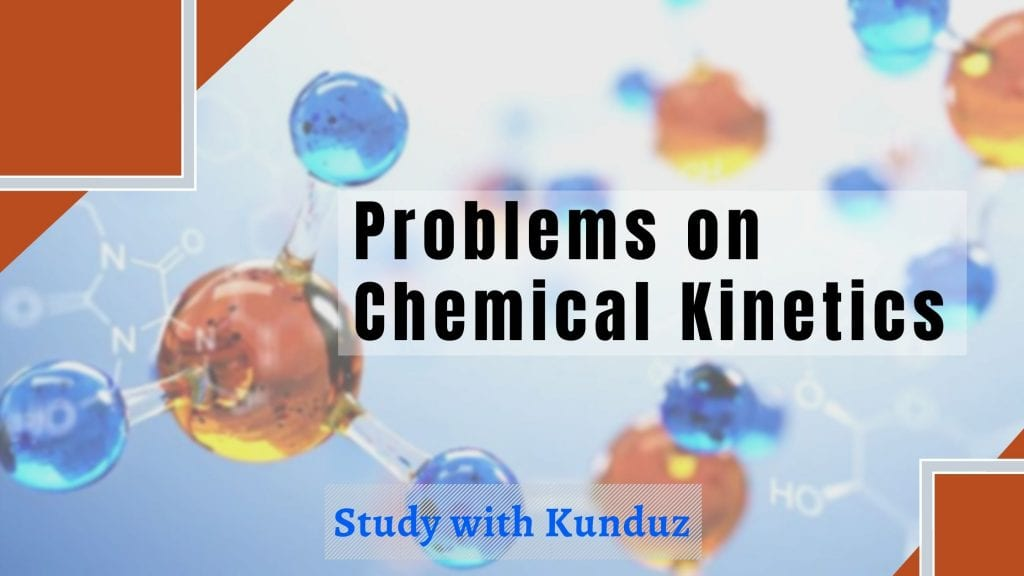Study Material

# Chemical Kinetics Practice Problems – with answers

Here you have 20 very important questions on chemical kinetics, which are good for practice as well as for revision. All the types of questions as covered.

4 minutes long
Posted by Mahak Jain, 1/11/2020Hesap Oluştur

Got stuck on homework? Get your step-by-step solutions from real tutors in minutes! 24/7. Unlimited.

To crack difficult exams like JEE and NEET and also to perform good in boards, a lot of practice is required. Here you have some questions on the topic chemical kinetics.

## Practice questions on chemical kinetics

#### 1

In reaction 2H2O2 → 2H2O + O2, rate of formation of O2 is 2.6 mol/L×sec then rate of disappearance of H2O2 is-

1. 2.6 mol/l×sec
2. 1.3 mol/l×sec
3. 5.2 mol/l×sec
4. 4.6 mol/l×sec

#### 2

For reaction A → A(g) + ½ C (g) at t=0 total pressure is 8atm, at time t=10sec total pressure of reaction is 10atm.calculate the rate of reactant A in atm×sec-1 is ?

1. 0.2
2. 0.4
3. 1.0
4. 1.8

#### 3

For reaction A + B → product, concentration of A and B with rate of reaction changes as follow

A             B              ROR

1.      0.02        0.08           1×10^-2

2.      0.04        0.08           2×10^-2

3.      0.02         0.16          4×10^-2   Order of reaction is-

1. 3
2. 2
3. 1
4. 4

#### 4

Activation energy of a chemical reaction can be determined by

1. Determining the rate constant at standard temperature
2. Evaluating the rate constants at two different temperatures
3. Determining probability of collision
4. Evaluating velocity of reaction at two different temperature

#### 5

A reaction follows zero order reaction. concentration of reactant is change as follow

In every 3 minute

20 →15 → X → Y , value of X and Y is (time interval is same 3 minute)-

1. 7.5 & 3.75
2. 10  & 5
3. 7.5 & 5
4. 10 & 3.75

#### 6

For a first order reaction A→B, the reaction rate at reactant concentration of 0.01 M is found to be 2.0×10−5 molL−1 s−1 . The half-life period of the reaction is:

1. 220 sec
2. 30 sec
3. 300 sec
4. 347 sec

#### 7

In zero order reaction slop of t1/2 v/s a is (a is initial contraction of reactant)-

1. 1/2K
2. 2k
3. 1/K
4. K

#### 8

A substance ‘A’ decompose by a first order reaction starting initially with [A] = 2.00M and after 200min, [A] becomes 0.15M. For this reaction t1/2 is

1. 53.72 min
2. 50.49 min
3. 48.45 min
4. 46.45 min

#### 9

Which is not correct for catalytic affect on reaction?

1. Catalyst does not change the equilibrium constant of reaction
2. Catalyst increases Gibbs Energy
3. It affect forward as well as backward at same extent
4. It decreases activation energy of reaction

Correct answer : 2. Gibbs Energy does not alter.

#### 10

Units of rate constant of first and zero order reactions in terms of molarity M units are respectively.

1. sec-1, Msec-1
2. sec-1, M
3. Msec-1, sec-1
4. M, sec-1

#### 11

A reaction follow zero order, slop of graph between t1/2 and concentration of reactant is 45°. Calculate the rate constant for reaction.

1. 0.5 mol/lit×sec
2. 2.0 mol/lit×sec
3. 1.414 mol/lit×sec
4. 1.0 mol/lit×sec

#### 12

For a first order reaction half life is 30 minute. The value of t99% is ?

1. 100 minutes
2. 200 minutes
3. 150 minutes
4. 250 minutes

#### 13

Which of the following is not correct(first order reaction) ?

1. t75% = 2 × t50%
2. t87.5% = 3 × t50%
3. t90% = 3.33 × t50%
4. t99% = 4 × t50%

#### 14

A first order reaction take 8 minutes to complete 90% reaction. Time taken in completing 99% is-

1. 16 minutes
2. 12 minutes
3. 14 minutes
4. 10 minutes

Correct answer : 1; t99% = 2×t90%

#### 15

The temperature dependence of rate constant (k) of a chemical reaction is written in terms of Arrhenius equation k=Ae−E/RT . Activation energy (Ea) of the reaction can be calculated by plotting-

1. K v/s T
2. logk v/s 1/T
3. K v/s 1/logT
4. logK v/s  1/logT

#### 16

A hypothetical reaction A2 + B2 → 2AB follow the following mechanism

A2 <__>A + A (fast)

A + B2 → AB + B (slow)

A + B → AB   (fast)

The order of the overall reaction is-

1. 2
2. 0
3. 1
4. 3/2

#### 17

For a reaction value of rate constant k is 2.4 × 10-6 mole/lit×sec. The reaction is-

1. Zero order
2. First order
3. Second order
4. None

#### 18

For A(g) + B(g) → C(g) + D(g), If initial concentration of A and B are increased by factor 2 and 4 respectively and R = k [A][B]1/2, then the initial rate is changed by factor

1. 2
2. 4
3. 6
4. Remain constant

#### 19

First order reaction completes 75% in 100 minutes. How long will it take for 87.5% completion?

1. 200 minutes
2. 175 minutes
3. 150 minutes
4. 125 minutes

#### 20

For an exothermic reaction :

1. Ea > ΔH
2. Ea < ΔH
3. Ea = ΔH
4. None of the above